# Ex.5.1 Q2 Introduction to Euclids Geometry Solution - NCERT Maths Class 9

Go back to  'Ex.5.1'

## Question

Give a definition for each of the following terms. Are there other terms that need to be defined first? What are they, and how might you define them?

(i) Parallel lines

(ii) Perpendicular lines

(iii) Line Segment

(v) Square

Video Solution
Introduction To Euclids Geometry
Ex 5.1 | Question 2

## Text Solution

Steps:

We have to define ‘Ray’, ‘Straight line’ and a ‘point’.

Ray: A part of a line, which starts at a point (Here A)and goes off in a particular direction to infinity possibly through a second point(B in this case).Straight Line: The basic concept about a line is that it should be straight and it can be extended infinitely in both the directions.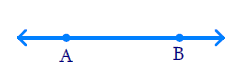Point: A small dot made by a sharp pencil on a sheet paper gives an idea about a point. A point has no dimension, it has only a position.

Perpendicular Distance: Shortest distance between a point and a line.

(i) Parallel lines:

• If the perpendicular distance between two lines is always constant, then these are called parallel lines.
• If the lengths of the common perpendiculars at different points on the lines are same, then these lines are called parallel lines.
• In other words, the lines which never intersect each other are called parallel lines.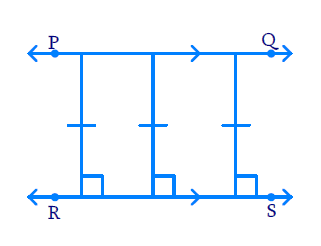(ii) Perpendicular lines: If the angle between two lines is equal to , then these lines are perpendicular to each other.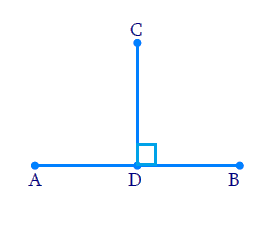\begin{align} { C D \perp A B } \\ { A B \perp C D } \end{align}

(iii) Line segment: A terminated line is called line segment. It has two end points [A and B in this case.].(iv) Radius of a circle: The distance from the center to any point on the circle is called radius of the circle.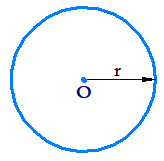(v) Square: A square is a regular quadrilateral which means that it has four equal sides and four right angles.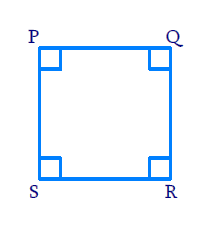Learn from the best math teachers and top your exams

• Live one on one classroom and doubt clearing
• Practice worksheets in and after class for conceptual clarity
• Personalized curriculum to keep up with school